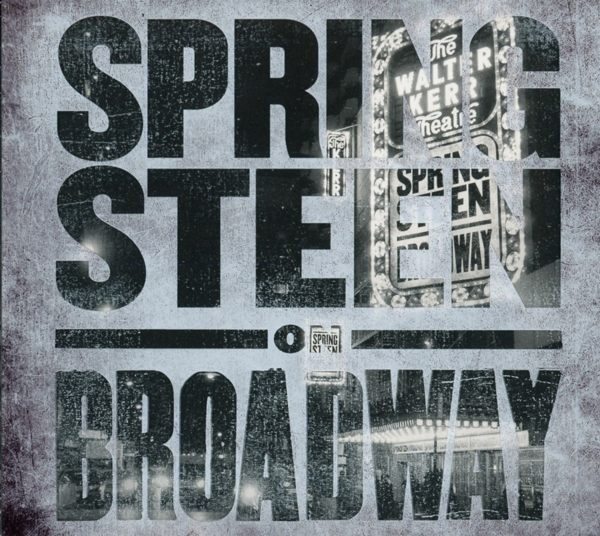Filters

# Springsteen On Broadway - Bruce Springsteen19,99 €
Genres:
Medium:
Tracklist:
Growin' Up (Introduction) (00:02:04)
Growin' Up (00:12:00)
My Hometown (Introduction) (00:07:21)
My Hometown (00:04:00)
My Father's House (Introduction) (00:04:31)
My Father's House (00:06:23)
The Wish (Introduction) (00:06:10)
The Wish (00:04:25)
The Promised Land (Introduction) (00:11:35)
The Promised Land (00:04:00)
Born In the U.S.A. (Introduction) (00:08:06)
Born In the U.S.A. (00:04:45)
Tenth Avenue Freeze-Out (Introduction) (00:01:10)
Tenth Avenue Freeze-Out (00:08:00)
Tougher Than the Rest (Introduction) (00:01:28)
Tougher Than the Rest (00:04:30)
Brilliant Disguise (Introduction) (00:01:43)
Brilliant Disguise (00:04:47)
Long Time Comin' (Introduction) (00:03:09)
Long Time Comin' (00:03:54)
The Ghost of Tom Joad (Introduction) (00:03:31)
The Ghost of Tom Joad (00:04:36)
The Rising (00:04:33)
Dancing In the Dark (Introduction) (00:02:50)
Dancing In the Dark (00:04:10)
Land of Hope and Dreams (00:03:58)
Born to Run (Introduction) (00:07:35)
Born to Run (00:05:10)